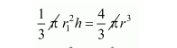# If a cone and a sphere have equal radii and equal volumes.`
Question:

If a cone and a sphere have equal radii and equal volumes. What is the ratio of the diameter of the sphere to the height of the cone?

Solution:

Given that,

A cone and a sphere have equal radii and equal volume

i.e., volume of cone = volume of sphere$r^{2} h=4 r^{3}$

$h=4 r$

$h=(2 r) \times 2$

$\frac{h}{2 r}=\frac{2}{1}$

$\frac{h}{2}=\frac{2}{1} \quad($ diameterd $=2 r)$

$h: d=2: 1$ or $d: h=1: 2$